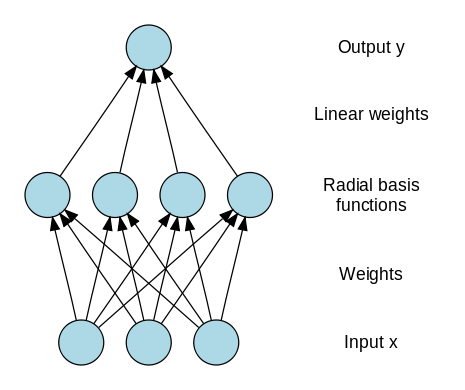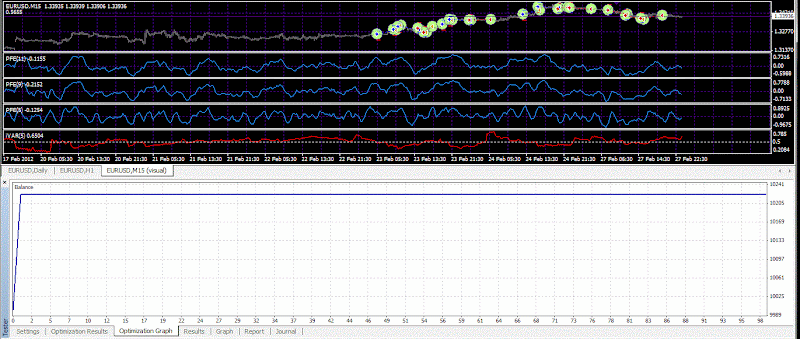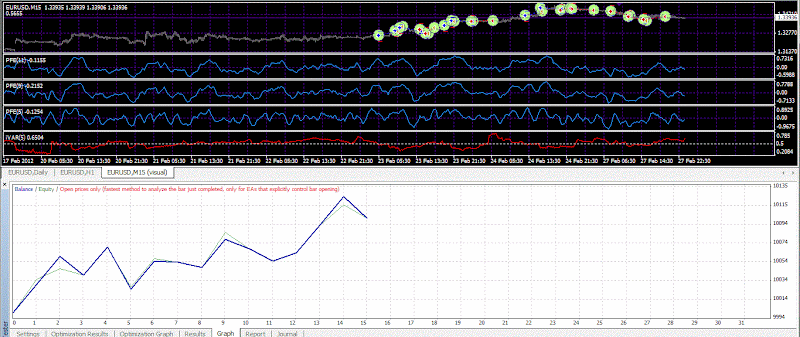## Spinal Implant v2 with Gaussian functionОт 23 септември 2011

Here on this mod I use a different and more classical neural net architecture for the perceptron.

Each input is feeding each neuron. And the transfer function is the gaussian function.

make an update of the perceptron function by adding cross relationships. This is for the gaussian transfer function.

In the original by going straight from imput to transfer function to output, what we do is a decision making tool.

However allowing as in the classical neural net architecture each imput feeds the neurone, in that way we allow th neural net to find some deeper characteristics of the price time series.

This is the modified code of the perceptron.

double perceptron()

{
double w1 = MathExp(-x1*x1/2);
double w2 = MathExp(-x2*x2/2);
double w3 = MathExp(-x3*x3/2);
double w4 = MathExp(-x4*x4/2);

double p1 = iCustom(NULL,Symbol(),"PFE",x5,true,5,0,0);
double p2 = iCustom(NULL,Symbol(),"PFE",x6,true,5,0,0);
double p3 = iCustom(NULL,Symbol(),"PFE",x7,true,5,0,0);
double p4 = iCustom(NULL,Symbol(),"PFE",x8,true,5,0,0);

return(w1*w2*w3*w4* p1 + w1*w2*w3*w4* p2 + w1*w2*w3*w4*p3 + w1*w2*w3*w4* p4);
}

• JohnLast 3009 days ago

I think there is something wrong with the code because on the back testing it open some trades when the ivar is bigger than 0.5 and my condition is to open trades only if Ivar is loever than 0.8 for the last two bars.

• JohnLast 3009 days ago

I removed one of the inputs, making 3 PFE instead of 4, and it works the way it has to.

I think that maybe it is metatrader inherent problem.

Regarding the architecture there is a similar commercial project that served as additional idea. It is from the neural package of neuroshell software.

In this package the neural net architecture is limited to two neurones, as a good practice. In our EA we use four neurones.

In the neuroshell software the number of inputs is from 2 to 6 with 2 hidden neurones.

• JohnLast 3008 days ago

One is using 4 inputs  but 2 neurones only.

The other is using 3 inputs and 4 neurones.

The perceptrone with 2 neurones is usefull when I want to ooptimize the Perceptron togwther with other parameters. For example the take profit and the stop loss.

Then I can take those two parameters fixed and use another perceptron with 4 neurones in order to have more detailed solution.

On this example when I found a fixed stop loss and take profit the optimization plot started to look like this. This was strange for me, as I am not used to such plots. The out of sample tests were very satisfying too.

The net was trained from 16.01.2012 to 22.02.2012. The out of sample is from 23.02.2012 until today 28.02.2012.

I use as ivar filter < 0.5 for tha last two consecutive bars.От 08 ноември 2011От 08 ноември 2011
• JohnLast 3008 days ago

I added the two other mods. I vary the neural net architecture. In the name is their architecture.

3inputs4neurones

4inputs2neurones

4inputs4neurones (this one has a little bit strange behaviour as mentionned above)

• jaguar1637 3008 days ago

Yep John

I was thinking of a motor composed by 3 perceptrons

1) Perceptron ((Close[i] - Open[i]) * iOBV(NULL, 0, PRICE_CLOSE, i) ) for Trend determination
2) Perceptron Stochastic for Momentum determination
3) Perceptron PFE for Direction determination

double Logic(double ptrend)
{ if(MathExp(ptrend)+MathExp(-ptrend) >0)
return( (MathExp(ptrend)-MathExp(-ptrend))/(MathExp(ptrend)+MathExp(-ptrend)) );
else
return(0);
}

double perceptronspeed()
{
double w1 = -Logic(x1*x1/2);
double w2 = -Logic(x2*x2/2);
double w3 = -Logic(x3*x3/2);
double w4 = -Logic(x4*x4/2);

double p1 = iCustom(NULL,Symbol(),"PFE",x5,true,5,0,0);
double p2 = iCustom(NULL,Symbol(),"PFE",x6,true,5,0,0);
double p3 = iCustom(NULL,Symbol(),"PFE",x7,true,5,0,0);
double p4 = iCustom(NULL,Symbol(),"PFE",x8,true,5,0,0);

return(w1 * p1 + w2 * p2 + w3 * p3 + w4*p4);
}

I will put the result in files attachment

• JohnLast 3008 days ago

Yes of course it is a nice idea you can use one perceptron for trend and another for momentum.

How looks like this activation function, where it comes from, or it is jaguar activation function ;).

In fact there are many, many possibilities.

• jaguar1637 3008 days ago

Check also, the new indicator based on 3 perceptrons and the expert advisor based on the same idea ;)

• JohnLast 3008 days ago

Thanks a lot I will check tomorow here it is almost two in the morning, bonne nuit.

• virux84 2600 days ago

any news on those ideas?

• JohnLast 2600 days ago

We had many mods passing from traditional activation functions to 3d dimensional activation functions.

The best in my opinion the logistic map as a learning tool.

Here is an article from me explaining in more detail the idea behind this.

Here is an example of an EA using the idea. The entropy is much faster to optimize than the iVAR.